# Team:WHU-China/modelingtp

(Redirected from Team:WHU-China/modeling)

# 1. Overview

For a pdf version of the tandem promoter modeling part,click hereThis model aims at predicting the final output of a tandem-repeat promoter system, which constitutes of repeated identical sub-promoter. The key idea of the model is that the strength of a promoter system is proportional to the probability of at least one RNA Polymerase (mentioned as RNAP latter) binding on the promoter.

# 2. Symbol table, Assumption and reasons.

 Definition Relative Strength The relative strength of certain promoter is defined by let the strength of Anderson promoter BBa_J23100 equals to one (in E.coli), and adjust the strength of other promoters accordingly. (http://parts.igem.org/Promoters/Catalog/Anderson) Normalized Strength The normalized strength of certain promoter is calculated by dividing the strength of the promoter by the highest promoter strength in the host. The highest promoter strength can be reached by creating artificial tandem promoter constitutes of the strongest known promoter. Symbol [ ] The symbol of concentration, i.e. [Protein] means the concentration of the protein ptot / y The probability of at least one RNAP(with all of its subunit) binding on the tandem promoter. It also means the normalized strength of the promoter. n / x The number of sub-promoters in the tandem promoter system. u Number of copies of a tandem promoter in a cell ξ Strength constant, equals to the strongest expression level possible (units in fluorenes normalized by a internal reference). V The volume of a cell pi The probability of a RNAP(with all of its subunit) form a RNAP-with complex with the ith sub-promoter in the tandem promoter system. qi qi=1-pi, the probability of a RNAP not binding to the ith sub-promoter j Cooperative factor α Transcription rate constant λ mRNA degradation constant v Translation rate constant k Protein degradation constant RNAP RNA Polymerase ODE Ordinary Differential Equation RP / RPc RNAP-Promoter complex, inactive complex RPi Intermediate complex RPo Open complex
Table 1. Symbol table of TP Model

• 1.It’s assumed that the promoter strength is measured in the same species, with identical environment and growing stage. This ensures that the concentration of all subunits of RNAP, all subunits of ribosome, all RNA degradation enzymes, all kind of proteases and all transportation protein are almost the same.
• 2.In all measurement, the contexts of the promoters remain the same. i.e. same RBS, terminator, protein sequence, up stream element, down stream element and DNA supercoiling.
• 3.All transcriptional factors are not considered in this version of the model, but can be included in the model with some modification to the equations.
• 4.The promoter region is accessible for RNAP(and all kinds of its subunits), which means it’s not in heterochromatin region or any other condition that hamper a normal RNAP-DNA interaction.
• 5.The probability of RNAP binding on the region between two sub-promoter within the tandem promoter system is neglected. As it contributes too little to final ptot.
• 6.The RNAP-DNA binding is assumed to stay on equilibrium in the model. This is reasonable because the open complex formation is a slow rate limiting step of transcription. So in the time scale of open complex formation, RNAP-DNA binding can always reach its equilibrium in neglectable time. It’s also observed that the inactive RNAP-DNA complex can be detected on the DNA.
• (*The following assumption is adopted by the commonly used thermodynamic based model , but it’s challenged in the later part of the model. We will first keep this assumption to derive the model, and modified the model for conditions that this assumption do not work. The weakness of this assumption is discussed in detail in here and here)
• 7.The probability (the speed) of RPc transforming to RPo is identical to all promoter, i.e. The strength of a promoter is merely related with the probability of RNAP binding to it. it enable us to calculate the promoter strength from the probability of RNAP binding to the promoter.

# 3. Modeling result

We found that the strength of a tandem promoter system can be interpreted by a simple equation: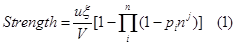Where qi is the probability of a RNAP(with all of its subunit) not forming a RNAP-with complex with the ith sub-promoter, n the number of sub-promoters, j the coordinative factor, and ξ the strength constant.

If we define the highest possible expression level of a promoter in certain species is 1. Then the equation 1 become normalized.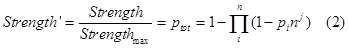This model explains 99% of the tandem promoter strength variation caused by number of sub-promoters.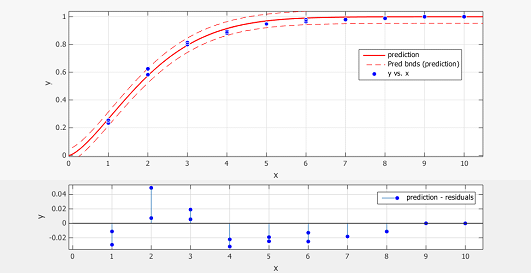Figure 1.Prediction vs. Data plot and residual plot
Y-axis shows the normalized promoter strength, X-axis the number of sub-promoters The blue dot is data extracted from of ref. fig.2 at14h and 25h, the red line is the prediction made by the model, the red dotted line is the 95% confidence bound.
The model also successfully predict the strength of J23102- 23102 (BBa_K1081002) and J23106-23106 (BBa_K1081005) tandem promoters, with error less than 10%.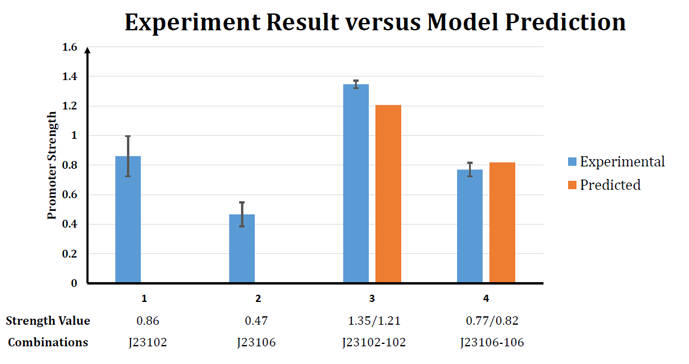Figure 2. Experiment result versus Model prediction

# 4.Model derivation

The promoter strength may be influenced by various factors. We need to simplify the system into some reasonable toy model by wiping out all relatively trivial factor.

4.1 Expression level Measurement
We use the fluorescence strength to indicate the strength of the promoter. Because when the exciting light is fixed, the fluorescence is proportional to the concentration of FP. And FP can be lighted up in a short time after they are synthesis.

4.2 Translation and transcription
According to the Central Dogma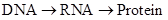So we can write down the following ODE, which is similar to the equations in .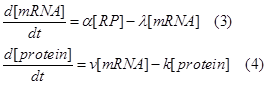Where α means the mRNA producing constant, λ the mRNA degradation constant, v the protein synthesizing, k the protein degradation constant, and [RP] is the concentration of RNAP-promoter promoter.
In equation 4, the protein increasing speed is determined by [mRNA] and v. With same RBS, v relates to the efficiency and concentration of ribosome and concentration of amino acids in the cell, which can be considered identical under the experiment condition of comparing different promoter. The protein degradation speed is determined by [protein] and k. k relates to protease system in the cell, which can also be considered as identical in measurements between different promoter.

In equation 3, the mRNA increasing speed is determined by [RP] and α, and its degradation depends on [mRNA] and λ. Both α and λ can be treated as constant in the experimental condition of comparing different promoter. As α depends on the transcription initiation efficiency, which is assumed to be identical for any RNAP-DNA complex for simplicity. This is reasonable because if α varies, the difference of α can be incorporated in [RP] (and finally in pi, see latter derivation). Though this part of the equation varies from the equations in , it is justified by the phenomenon that when [RNAP] and [DNA] is hold in a constant, the UTP incorporation is a zero order reaction . And λ depends on the concentration of RNase which doesn’t varies in different promoter measurement.

Therefore, because we are interested in the steady state of the protein expression. We can set,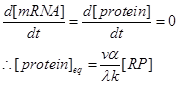We can consider [protein]eq as the indicator of the promoter strength, and let vα/ λk=ξ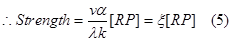So the strength of the promoter is directly related to the concentration of the RNAP-DNA complex of this promoter.

4.3 RNAP binding and transcription initiation
The open complex formation reaction is as follow.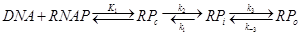Where RPc is the inactive complex, RPi is the intermediate complex and RPo the open complex. The reaction can be combined with Central Dogma to be: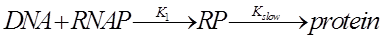Because K1 happens in a much smaller time scale. The probability of finding the polymerase on the promoter will be given by its equilibrium constant K1.

To evaluate the probability of polymerase binding (pi) we must sum the Boltzmann weights over all possible states of P polymerase molecules on DNA.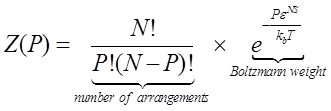This equation calculate the total Boltzmann weight of no RNAP binding to the target promoter, with N represent the number of non-specific sites on the DNA, P the effective RNAP number, ε^NS the non-specific binding energy, kb the Boltzmann constant and T the temperature.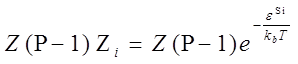This equation calculate the total Boltzmann weight of one RNAP binding to promoter i, with ε^Si means the specific binding energy of promoter i.
So the probability of a RNAP binding to promoter i is,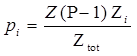With Ztot represent the sum of all Boltzmann weight of all different condition.
So the probability of RNAP binding to both promoter i and j is,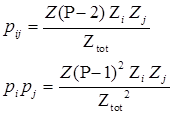when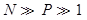we have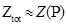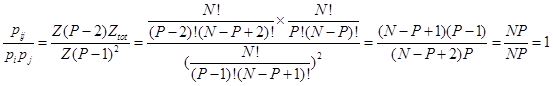So the probability of RNAP binding to two promoter at the same time equals to the product of the probabilities of RNAP binding to the two promoter respectively. i.e.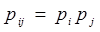As only one RNAP is needed to initiate the transcription in a tandem promoter system (the other RNAP will be blocked by the RNAP closest to the transcription initiation point). So the probability of at least one RNAP binding to the promoter is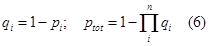For a kind of promoter with u copies in a cell (all separated and function independently)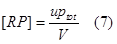The strength of a promoter is, according to equation 5.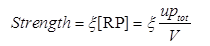the maximum strength possible can be reached when ptot=1,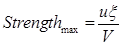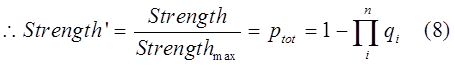However, the prediction fail to explain the data.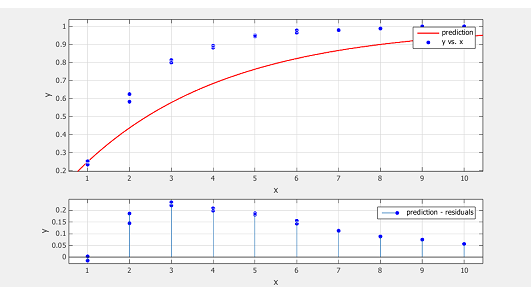Figure 3. Prediction vs. Data and residual plot of the simpler model
Y-axis shows the normalized promoter strength, X-axis the number of sub-promoters The blue dot is data extracted from of ref. fig.2 at14h and 25h, the red line is the prediction made by the model
The data increase in y much quicker than our prediction, which indicate there will be some kind of cooperation among sub-promoters. This results in pij>pipj. The cooperation can be explained by the fact that when one RPo formed, it will “melt” the DNA duplex into two single strain. This DNA untwisting, unwinding and melting make the RNAP-DNA complex in the vicinity easier to transform from RPc to RPo. Therefore variation in α can no longer be ignored.

So we should add a adjust term(the cooperation factor) into equation 8. Therefore equation 2 comes out, with nj as the cooperative factor.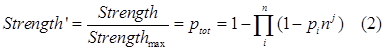As we’ve showed in figure 1. This model successfully captures the essence of tandem promoter system.

# 5.Discussion

Because it failed to capture the interaction between sub-promoters, the flawed (but widely adopted) assumption 7 was proved inapplicable in tandem-repeat promoter strength prediction. Our data further showed that the it can not be employed to general tandem promoter condition.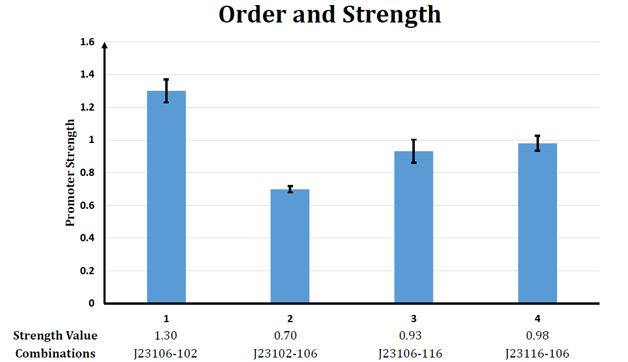Figure 4. The relative strength of four tandem promoter
Under assumption 7, the order of sub-promoters has nothing to do with the final output of the promoter. But obviously, though the strength of promoter J23116-106 and J23106-116 have no much difference, the strength of promoter J23102-106 differs greatly from the strength of promoter J23106-102.

All these data reveal that there are various significant interaction between sub-promoters. And the α of different promoters varies a lot (Thus results in the giant difference between the strength of promoter J23102-106 and the strength of promoter J23106-102).

The reason why the model works well in tandem-repeat promoter are:
1. The α is identical for all sub-promoters.
2. The cooperative factor successfully captures the interaction between sub-promoters.

So, it’s understandable why the model cannot be easily modified to predict the strength of any randem tandem promoter. Because,
1. The α of different sub-promoters may vary.
2. The interaction between different promoter may vary a lot. (Thus results in the difference between J23102-106/J23106-102 and J23116-106/J23106-116)

There is another two minor problem of the model.
1. The cooperative factor has no solid biological ground (it’s even a boundless function when x approach infinite). The more prudent way will be choosing a sigmoid function rather than nj as the cooperative factor. But that will make the model more complex and hard to employ when people just have scarce data about their promoter (easy over-fitting). So we decide to keep it in this simpler and efficient form.
2. The difference of translation efficiency caused by the length variation of mRNA 5’-UTR is ignored in the model. This will not undermine the accuracy of the model, because the influence of the length of 5’-UTR before RBS is trivia when the length is short, and the tandem promoter is often shorter than 100bp. It’s reported that changing the operon order of GGPP synthase and taxadiene synthase affect taxadiene synthase expression by 20% (GGPP synthase plus its RBS is ~1kb)

# 6.User Guideline

To employ the model, the user need to assign the pi for each kind of promoter that will be used to construct the tandem promoter.

The simplest way to achieve it is as follow.
1)Using fluorescence protein to indicate the expression level of each promoter or promoter association, optional (normalize it by a internal reference just as we used a RFP in our experiment).
2)To measure the strongest expression level possible in the species. Using a known strongest promoter to construct a tandem promoter that made of 5 repeats of the promoter, to see the strongest expression level.
3)Normalizing other promoter’s expression level by the strongest expression level, which result in the pi of each promoter. As follow.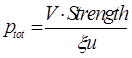4)using equation 2 to predict the ptot of the designed tandem promoter, with an empirical cooperative factor j=0.4.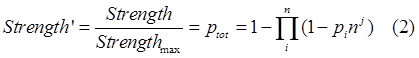In this way, the error of the prediction should be less than 4% of the maximum expression rate, as our data showed before.
If the data allow, the user can carry out fit with a variable j, which may varies in different species and cell condition.

# Reference:

1.Bintu, Lacramioara, et al. "Transcriptional regulation by the numbers: models." Current opinion in genetics & development 15.2 (2005): 116-124.
2.Buc, Henri, and William R. McClure. "Kinetics of open complex formation between Escherichia coli RNA polymerase and the lac UV5 promoter. Evidence for a sequential mechanism involving three steps." Biochemistry24.11 (1985): 2712-2723.
3.DeHaseth, Pieter L., and John D. Helmann. "Open complex formation by Escherichia coli RNA polymerase: the mechanism of polymerase‐induced strand separation of double helical DNA." Molecular microbiology 16.5 (1995): 817-824.
4.Li, Mingji, et al. "A strategy of gene overexpression based on tandem repetitive promoters in Escherichia coli." Microb Cell Fact 11 (2012): 19.
5.Buchler, Nicolas E., Ulrich Gerland, and Terence Hwa. "Nonlinear protein degradation and the function of genetic circuits." Proceedings of the National Academy of Sciences of the United States of America 102.27 (2005): 9559-9564.
6.Alon, Uri. Introduction to Systems Biology: And the Design Principles of Biological Networks. Vol. 10. CRC press, 2007. Page 6.
7.Nishizaki, Tomoko, et al. "Metabolic engineering of carotenoid biosynthesis in Escherichia coli by ordered gene assembly in Bacillus subtilis." Applied and environmental microbiology 73.4 (2007): 1355-1361.